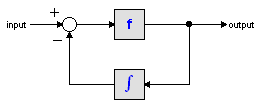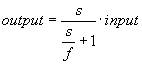﻿ 20-sim webhelp > Library > Iconic Diagrams > Mechanical > Translation > Sensors > accelerationsensor-relative

accelerationsensor-relative

Library

Iconic Diagrams\Mechanical\Translation\Sensors

Use

Domains: Continuous. Size: 1-D. Kind: Iconic Diagrams (Translation), Block Diagrams.

Description

This model describes an acceleration sensor which derives an acceleration output out of a velocity difference (between high and low terminals) by differentiation. Differentiation is performed by a state variable filter:The S-domain function of this filter is equal to:where f is the cut-off frequency. For very high values of f, the output becomes the pure derivative of the input. High values of f, however, increase simulations times. A good trade-off is a starting value of 1e5.

The equations of this model are:

a = d( p_high.v - p_low.v)/dt;

p_low.F = p_high.F = indifferent;

Interface

 Ports Description p_high, p_low Both terminals of the Translational port p. Causality fixed force out Output a Acceleration (measured as the difference between both terminals) [m/s2] Parameters f a_initial Cut-off frequency of the differentiation [Hz]. Initial acceleration [m/s].